Next: D Integration of Fermi Up: C Solutions to Schrödinger's Previous: C.2 The Finite Potential

# C.3 The Rectangular Potential Barrier

We calculate the tunnel transmission coefficient for an electron wave packet which hits the rectangular potential barrier with height E0 and width d (see Fig. C.3).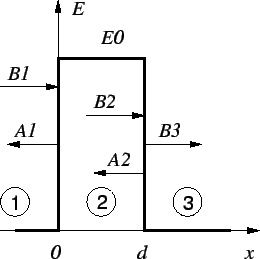Starting with an Ansatz for the three regions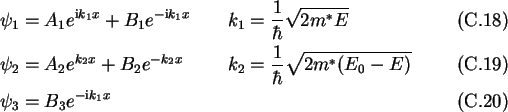and the boundary conditions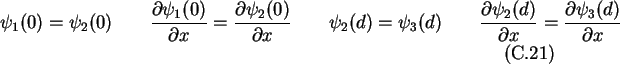one obtains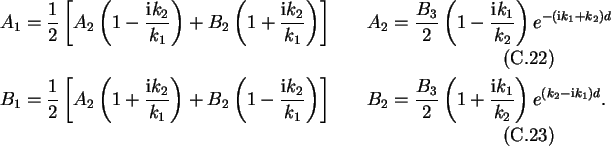The ratio of incoming and transmitted probability amplitude is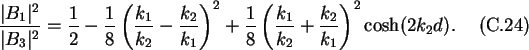The transmission probability depends exponentially on thickness and height of the barrier.

Christoph Wasshuber